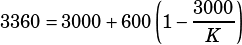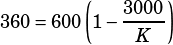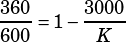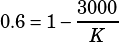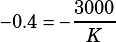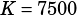Please work problem # 38 in Test 2, Section 4.

This one is all about following the formula and knowing where to plug the given numbers in. Here’s the formula: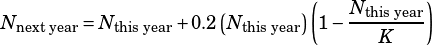The N values are obvious: they represent the number of plants the botanist will have this year and next year. K is less obvious from the formula, but the description of the formula tells you what it is: K is the number of plants the environment can support. That, it turns out, is what question #38 is asking you to solve for. To go from 3000 plants this year to 3360 next year, what must the value of K be?